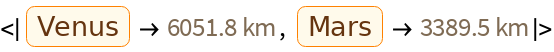#Function Repository Resource:

# TakeSmaller

Take values smaller than a threshold in a list or association

Contributed by: Wolfram Staff
 ResourceFunction["TakeSmaller"][list,val,n] gives the largest n elements in list that are smaller than val. ResourceFunction["TakeSmaller"][list→prop,val,n] gives the property prop for the n elements in list that are smaller than val. ResourceFunction["TakeSmaller"][val,n] represents an operator form of ResourceFunction["TakeSmaller"] that can be applied to an expression.

## Details and Options

ResourceFunction["TakeSmaller"] sorts by numerical magnitude.
ResourceFunction["TakeSmaller"] handles the same objects and properties as TakeSmallest.
ResourceFunction["TakeSmaller"] expects all objects to be comparable with one another.
ResourceFunction["TakeSmaller"][list,val,UpTo[n]] takes n elements, or as many as are available.
ResourceFunction["TakeSmaller"][assoc,val,n] gives an association of length n by taking the n largest values that are smaller then val in assoc, preserving their keys.
ResourceFunction["TakeSmaller"][val,n][list] is equivalent to ResourceFunction["TakeSmaller"][list,val,n].

## Examples

### Basic Examples

Take two numbers smaller than the specified value in a list:

 In:=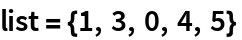Out=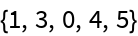In:=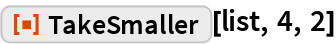Out=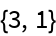Do the same using the operator form of TakeSmaller:

 In:=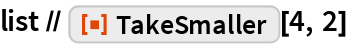Out=In:=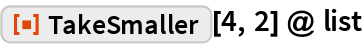Out=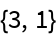Take the largest two values smaller than the specified threshold in an association:

 In:=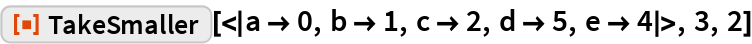Out=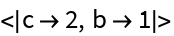Take the largest four numbers smaller than the specified value, or as many as are available:

 In:=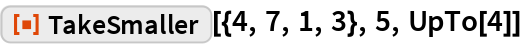Out=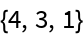Take all numbers with values under the threshold:

 In:=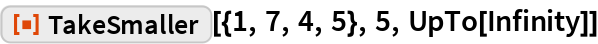Out=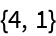Take two values in a list of Quantity objects:

 In:=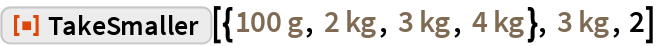Out=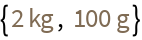### Scope

Get the two largest elements that are smaller than 23 in a list:

 In:=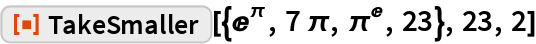Out=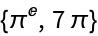Get the positions of the two largest elements smaller than 3:

 In:=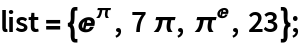In:=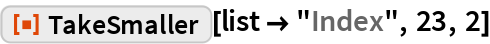Out=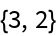Get the elements along with their positions:

 In:=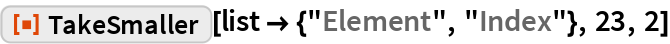Out=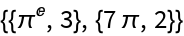Let positions come first in the result:

 In:=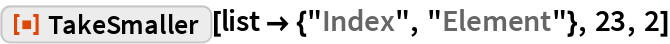Out=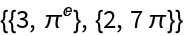Get associations containing the element and the position:

 In:=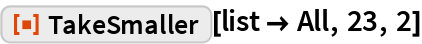Out=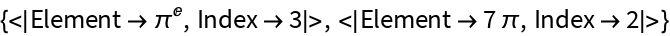Get the two largest planets with radii smaller than Earth's:

 In:=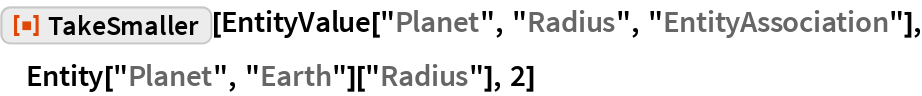Out=